# Prime Constellations By Matt Anderson 492011 Prime numbers

• Slides: 15Prime Constellations By Matt Anderson 4/9/2011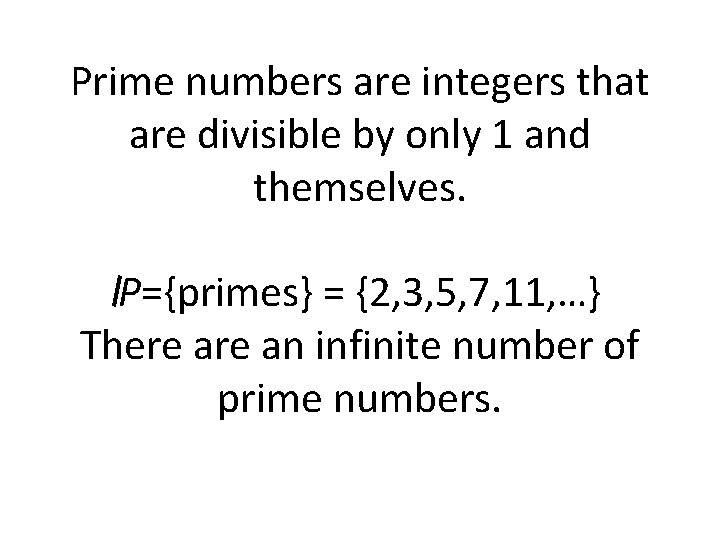Prime numbers are integers that are divisible by only 1 and themselves. P={primes} = {2, 3, 5, 7, 11, …} There an infinite number of prime numbers.Let π(x) be the prime counting function. π(x) counts the number of primes less than or equal to x. X π(x) 1 0 2 1 3 2 4 2 5 3 6 3 7 4 8 4 9 4The prime number theorem states that π(x) grows like x/Ln(x). Specifically,A prime k-tuple is an ordered set of values representing a repeatable pattern of prime numbers. Examples Instances (0, 2) twin primes (0, 4) cousin primes (0, 6) sexy primes (0, 2, 6) 3 -tuple (0, 4, 6) 3 -tuple (0, 2, 6, 8) 4 -tuple {3, 5}, {5, 7}, {11, 13} {3, 7}, {7, 11}, {13, 17} {5, 11}, {7, 13}, {11, 17} {3, 5, 11}, {5, 7, 13} {7, 11, 13}, {13, 17, 19} {5, 7, 11, 13}, {11, 13, 17, 19}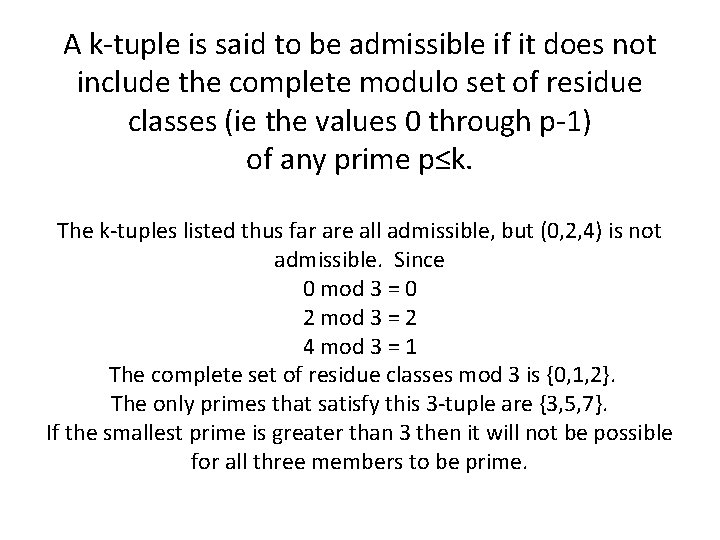A k-tuple is said to be admissible if it does not include the complete modulo set of residue classes (ie the values 0 through p-1) of any prime p≤k. The k-tuples listed thus far are all admissible, but (0, 2, 4) is not admissible. Since 0 mod 3 = 0 2 mod 3 = 2 4 mod 3 = 1 The complete set of residue classes mod 3 is {0, 1, 2}. The only primes that satisfy this 3 -tuple are {3, 5, 7}. If the smallest prime is greater than 3 then it will not be possible for all three members to be prime.An admissible prime k-tuple that is maximally dense is called a constellation with k primes. For n≥k, this will always produce consecutive primes. Where, n is the smallest prime in the constellation. Example: The constellation with 2 primes is (0, 2). Example 2: There are 2 constellations with 3 primes. They are (0, 2, 6) and (0, 4, 6).It is conjectured that there an infinite number of twin primes. Also, it is conjectured that there an infinite number of primes for every admissible k-tuple. Numerical evidence supports this conjecture.Let π m 1, m 2, …, mk (x) be the number of (k+1) tuples less than x For example, consider the 4 -tuple (0, 2, 6, 8) The smallest prime has the form 30 n+11. x π2, 6, 8(x) smallest examples 0 0 {5, 7, 11, 13} 5 1 {11, 13, 17, 19} 11 2 {191, 193, 197, 199} 191 3 {821, 823, 827, 829} 821 4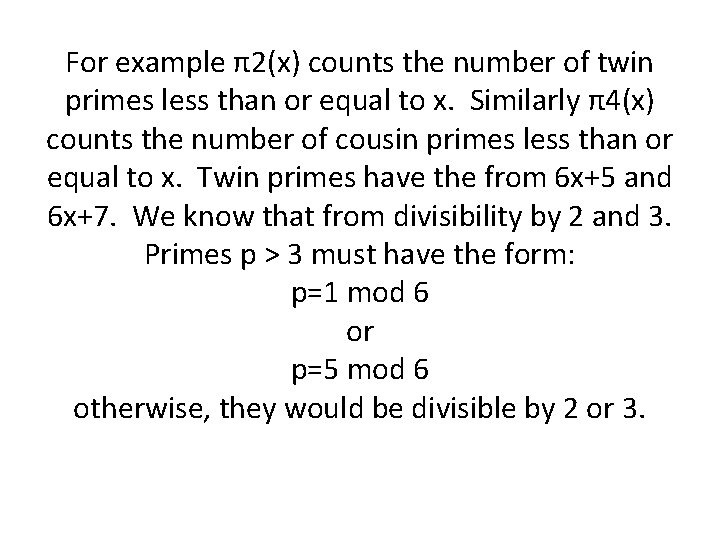For example π2(x) counts the number of twin primes less than or equal to x. Similarly π4(x) counts the number of cousin primes less than or equal to x. Twin primes have the from 6 x+5 and 6 x+7. We know that from divisibility by 2 and 3. Primes p > 3 must have the form: p=1 mod 6 or p=5 mod 6 otherwise, they would be divisible by 2 or 3.To find k-tuples, one must determine the values of a and b in p=ax+b for the smallest prime p in a constellation. One way to do this is by examining Ur# where r# (read r primorial) is the product of the first r primes and Ur# is the set of units mod r#. For example: U 2 = {1}. All prime numbers greater than 2 are odd. U 6 = {1, 5}. All primes > 3 have the form 6 k± 1 U 30 = {1, 7, 11, 13, 17, 19, 23, 29} r r# 1 2 2 6 3 30 4 210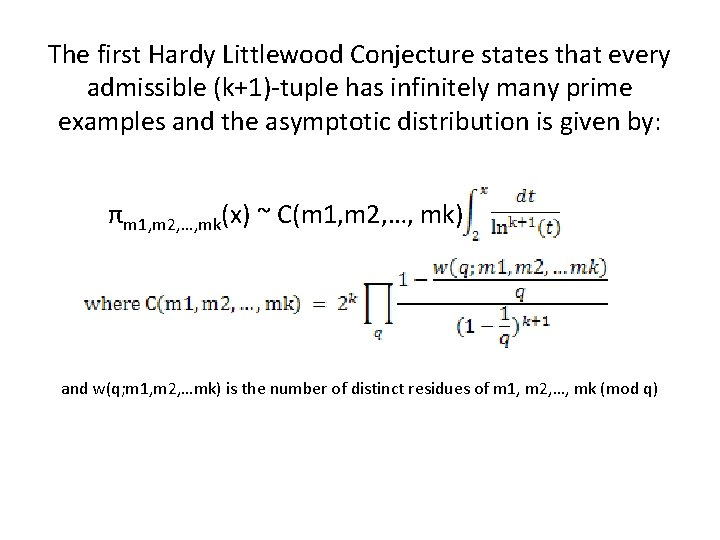The first Hardy Littlewood Conjecture states that every admissible (k+1)-tuple has infinitely many prime examples and the asymptotic distribution is given by: πm 1, m 2, …, mk(x) ~ C(m 1, m 2, …, mk) and w(q; m 1, m 2, …mk) is the number of distinct residues of m 1, m 2, …, mk (mod q)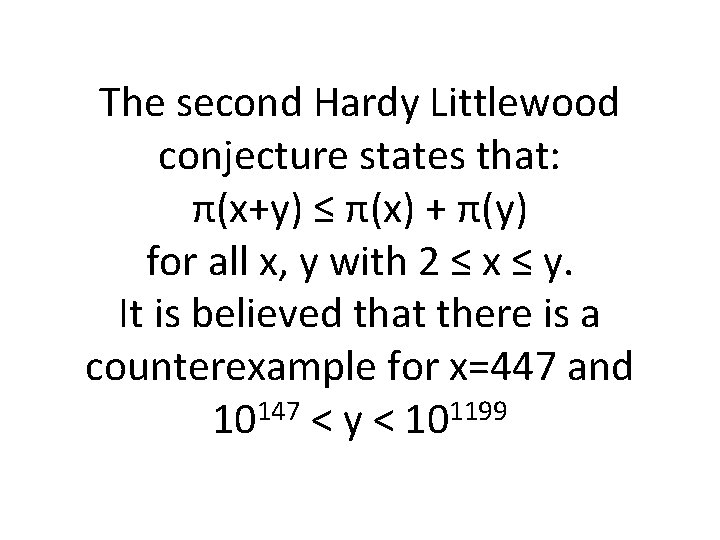The second Hardy Littlewood conjecture states that: π(x+y) ≤ π(x) + π(y) for all x, y with 2 ≤ x ≤ y. It is believed that there is a counterexample for x=447 and 147 1199 10 < y < 10Examples have been found for constellations of 2 to 23 primes. There are no known examples of constellations with 24 primes.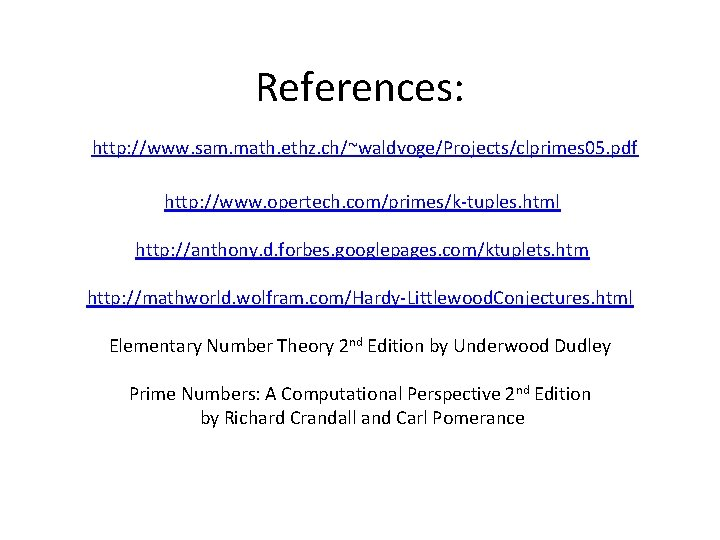References: http: //www. sam. math. ethz. ch/~waldvoge/Projects/clprimes 05. pdf http: //www. opertech. com/primes/k-tuples. html http: //anthony. d. forbes. googlepages. com/ktuplets. htm http: //mathworld. wolfram. com/Hardy-Littlewood. Conjectures. html Elementary Number Theory 2 nd Edition by Underwood Dudley Prime Numbers: A Computational Perspective 2 nd Edition by Richard Crandall and Carl Pomerance